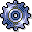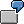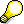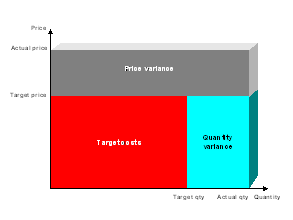Input Side VariancesUse

The input side consists of all debits and credits on the cost center or business process, other than credits due to activity allocations.

The input side compares actual costs with target costs. Input side variances include additional costs, reduced costs, and changed consumption of the individual cost elements, divided into fixed and variable portions. The input side variance categories include:

The variance categories of the input side are determined periodically for each cost element.

Features

Input price variances

Input price variance indicates changes in costs due to prices.

Variances caused by price and quantity differences are also assigned to input price variances.If you planned 10 labor hours worth 1000 USD for a cost element, and posted 10 hours worth 2000 USD in the actual, the price variance for the 2000 USD equals 1000 USD. Based on the plan data, 10 hours should cost 1000 USD. The difference between actual and plan data is due to higher prices (wages in this case) because there is no difference in activity quantity consumed.

The R/3 System calculates and posts the input price variances for primary postings by business transaction, as described in the Implementation Guide (IMG) under "Determining Primary Data Price Variances". You can display input price variances in the information system.

The R/3 System calculates the input price variances again if you specify quantities long with costs in the postings.

If the quantities are incomplete or missing, the R/3 System takes the input price variances from the posting rates of actual costs as calculated on a percentage basis during postings by business transaction. If no percentage rates exist, the R/3 System cannot calculate input price variances.

In target/actual comparisons, the input price variances are defined by the following formulas:

Input price variance = (Actual price – Plan price) X Actual input quantity

Fixed input price variance = (Fixed actual price – Fixed plan price) X Actual input quantityFor activity relationships using predistribution of fixed costs, the system uses the posted input price variances of the totals records (see: Predistribution of Fixed Costs).

Input quantity variance

Input quantity variance indicates under- and over-consumption for cost elements.

Variances arising from both price and quantity differences appear as input price variances.To support the activity type Machine Hours, you plan the consumption of 10 liters of lubricant in proportion to the activity quantity produced. For an operating rate of 110%, the actual lubricant consumption is 12 liters. The input quantity variance amounts to one liter. To produce the cost center activity, there was an over-consumption of one liter of lubricant when compared to the plan. Consumption based on the operating rate should be only 11 liters.

For target/actual comparisons, the quantity variances are defined by the following formulas:

Input quantity variance = (Actual input quantity – Target input quantity) X Plan price

Fixed input quantity variance = (Actual input quantity – Target input quantity) X Fixed plan price

Calculation of input quantity variances is possible only if complete consumption quantities exist in both target and in actual.For activity relationships using predistribution of fixed costs, the system uses the difference between target costs and actual costs, minus the input price variance, as the input quantity variance (see: Predistribution of Fixed Costs).Hints for Cost Center Accounting:

• To determine input price or input quantity variances, the SAP System requires consumption quantities as well as plan and actual costs. In this way variance calculation can determine cost and consumption influences and display them. It is often sufficient, however, to plan consumption quantities only for those cost elements that are subject to price fluctuations and also represent important costs. Enter a unit of measure in the master data for the given cost element. You can also use units of measure for which a conversion is possible. Additionally, if you select Record quantities in the cost element and cost center master data, the R/3 System issues a warning if you do not post quantities. The deciding factor for the separate calculation of quantity and price variances is the unit of measure.
• If recording consumption quantities is difficult or impossible, you can still determine input price variances. To do so, you must store percentage input price variances for individual cost elements during Customizing. The R/3 System uses these input price variances based on transaction for primary postings and for period-end closing during variance calculation. The system displays the resulting input quantity variance in the remaining input variance (see: For more information, see the IMG for Cost Center Accounting, under Actual Postings ® Variances ® Specify Primary Data for Input Price Variances).

Resource-usage variance

Resource-usage variance indicates changes in the plan consumption of cost elements. It occurs if you post an unplanned cost element in actual, or if no actual data exists for a plan cost element.

The resulting difference between plan and actual postings cannot be assigned to either input price or input quantity variance. The R/3 System has no basis for comparing plan and actual data postings. They are thus identified as variances in resource usage.You post plan costs of 100 USD on a cost center under cost element 400.000. You make the corresponding actual posting under cost element 410.000. Variance calculation identifies both entries as resource-usage variances equal to the amount of the posted costs.

The system determines resource-usage variances if either no controllable costs, or no target costs exist for a cost element.

In target/actual comparisons, the resource-usage variances are defined by the following formulas:

Resource-usage variance = Actual costs – Target costs – Input price variance

Fixed resource-usage variance = Fixed actual costs – Fixed target costs – Fixed input price variance

Remaining input variance

Remaining input variance includes all the variances occurring on the input side which cannot be assigned to any of the following categories:

• Input price variances
• Input quantity variance
• Resource-usage variance

This can be due to several causes:

• You planned cost elements and made actual postings, but did not record consumption quantities. The SAP R/3 System therefore cannot determine input price or input quantity variances.
• A user deactivated variance calculation for one of the listed categories. The resulting variance amount is assigned by the system to the remaining input variance.

For many organizations, remaining input variance is often the classic consumption variance (input quantity variance). Cost elements that react quickly to price changes are recorded by quantity, which ensures an exact calculation of input price and input quantity variances for these cost elements. For cost elements with little or no price sensitivity, the difference between actual and target costs is usually caused by greater consumption. This procedure reduces the requirements for entering plan and actual data, but lets you display results for individual cost elements.

If you specified in the variance variant that the system does not calculate input price, input quantity, or resource-usage variances, the system displays only the remaining input variance on the input side.

Input Side Variance Categories